# AP Chemistry : Molecular and Electronic Geometries

## Example Questions

### Example Question #1 : Molecular And Electronic Geometries

What is the molecular shape of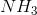?

Trigonal planar

Trigonal pyramidal

Trigonal bipyramidal

Tetrahedral

Octahedral

Trigonal pyramidal

Explanation:

NH3 is trigonal pyramidal because it has 4 electron domains, one of them being a lone pair of electrons and the other three being H atoms. When this is arranged in a three-dimensional space, it is trigonal pyramidal in shape.

### Example Question #11 : Molecular And Electronic Geometries

What is the bond angle in the following structure?

COH2

120
145
120 and 109.5
180
109.5
Explanation:

CO2H is trigonal planar, so the bond angles will be 120 only.

### Example Question #11 : Molecular And Electronic Geometries

What is/are the approximate bond angle(s) in the following molecule?

COH2

90, 109.5

90, 120

180

109.5

120

120

Explanation:

COH2:

:O:

||

H—C—H

This is a trigonal planar molecule, which only has bond angles of 120o

### Example Question #12 : Molecular And Electronic Geometries

Consider the following reactions that lead to the production of dichloromethane,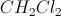: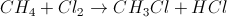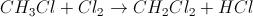What is the Cl-Cl bond angle of the product, dichloromethane?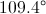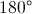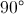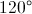Explanation:has four bonding regions and no lone pairs, so it has a tetrahedral geometry. The bond angles between all of the bonded atoms is.

### Example Question #33 : Molecules

What are the flourine-flourine bond angles in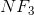?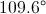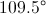Explanation:has three bonds and one lone pair, so it has a tetrahedral geometry with a trigonal pyramidal shape. Due to its shape, the bond angles are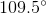.

### Example Question #131 : Compounds And Molecules

Which of the following molecules has the largest bond angle between atoms?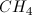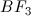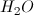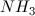Explanation:

In a compound, the atoms (and electron lone pairs) will be as far away from each other as possible due to the repulsive forces between electrons.

Methane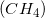will have a tetrahedral geometry with bond angles of 109.5o. Both ammonia and water have lone electron pairs, which will decrease the bond angle between atoms. Water will be bent, while ammonia will be trigonal pyramidal. Bent and trigonal pyramidal are both permutations of tetrahedral, with slightly decreased bond angles.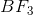does not have lone electron pairs and will have a trigonal planar geometry. This results in bond angles of 120o. As a result,has the largest bond angles out of the options.

### Example Question #35 : Molecules

What are the bond angles in a molecule of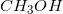?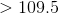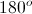The molecule has multiple bond angle measures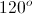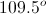Explanation:

The carbon in the molecule is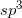hybridized and contains no lone pairs attached to it. This will give the molecule a tetrahedral geometry, standard of a central atom with four single bonds. Bond angles in a tetrahedral molecule are all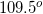.

### Example Question #11 : Molecular And Electronic Geometries

Rank the following compounds in order of increasing bond angle: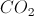,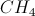,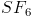,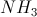,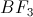If there are multiple bond angles in a single molecule, only consider the smaller bond angle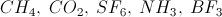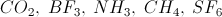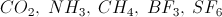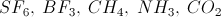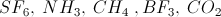Explanation:has two bonding regions and no lone pairs around the central atom, so it has a linear geometry, so its bond angle is.has four bonding regions and no lone pairs around the central atom, so it has a tetrahedral geometry with bond angles of.has six bonding regions and no lone pairs around the central atom, so it has an octahedral geometry with bond angles ofand. For the sake of ranking, we will only consider the smaller angle.has three bonding regions and one lone pair around the central atom, so it has a trigonal pyramidal geometry with bond angles of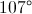.has three bonding regions and no lone pairs around the central atom, so it has a trigonal planar geometry with bond angles of.

### Example Question #131 : Compounds And Molecules

Consider the following reaction, called the Sabatier reaction: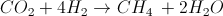What are the molecular geometries of the products?

Square planar and bent

Square planar and linear

Tetrahedral and tetrahedral

Tetrahedral and bent

Tetrahedral and linear

Tetrahedral and bent

Explanation:has four bonding regions and no lone pairs, so it has a tetrahedral geometry.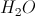has two bonding regions, but it also has two lone pairs, so it has a bent geometry.

### Example Question #11 : Molecular And Electronic Geometries

What are the shapes of the following compounds: (a) SF6 (b) XeF4 (c) NH3 (d) CH4
(e) PCl5?

square planar, trigonal bipyramidal, trigonal pyramidal, tetrahedral, octahedral

octahedral, square planar, trigonal pyramidal, tetrahedral, trigonal bipyramidal

trigonal bipyramidal, square planar, tetrahedral, octahedral, trigonal pyramidal

square planar, octahedral, trigonal pyramidal, tetrahedral, trigonal bipyramidal